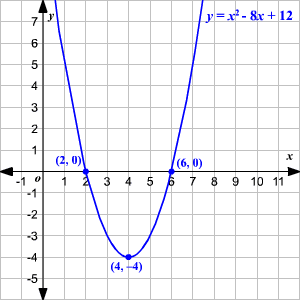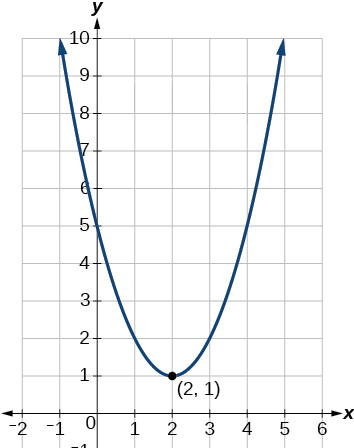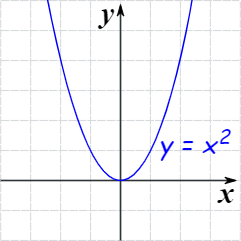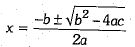Important Definitions & Formulas: Quadratic Equations

# Important Definitions & Formulas: Quadratic Equations Notes | Study Mathematics (Maths) Class 10 - Class 10

## Document Description: Important Definitions & Formulas: Quadratic Equations for Class 10 2022 is part of Mathematics (Maths) Class 10 preparation. The notes and questions for Important Definitions & Formulas: Quadratic Equations have been prepared according to the Class 10 exam syllabus. Information about Important Definitions & Formulas: Quadratic Equations covers topics like Standard Form of a Quadratic Equation, Word Problem Solved Examples and Important Definitions & Formulas: Quadratic Equations Example, for Class 10 2022 Exam. Find important definitions, questions, notes, meanings, examples, exercises and tests below for Important Definitions & Formulas: Quadratic Equations.

Introduction of Important Definitions & Formulas: Quadratic Equations in English is available as part of our Mathematics (Maths) Class 10 for Class 10 & Important Definitions & Formulas: Quadratic Equations in Hindi for Mathematics (Maths) Class 10 course. Download more important topics related with notes, lectures and mock test series for Class 10 Exam by signing up for free. Class 10: Important Definitions & Formulas: Quadratic Equations Notes | Study Mathematics (Maths) Class 10 - Class 10
 1 Crore+ students have signed up on EduRev. Have you?

Standard Form of a Quadratic Equation

The standard/general form of quadratic equation is ax2 + bx + c = 0 where,

a ≠ 0 and a, b, c are real numbers.

In this equation, the variable is ‘x’ and the degree of the equation is ‘2.’

Zeroes & Roots of the Quadratic Equation

The values of x for which a quadratic equation is satisfied are called the roots/zeroes of the quadratic equation.

If α is a root of the quadratic equation ax2+bx+c=0, then aα2+bα+c=0.

A quadratic equation can have two distinct real roots, two equal roots or real roots may not exist.

Graphically, the roots of a quadratic equation are the points where the graph of the quadratic polynomial cuts the x-axis.

Example: Consider y = x2 - 8x +12The graph of the above quadratic equation is intersecting the x-axis at two points. So, the given quadratic equation has two distinct real roots.The above quadratic equation is not intersecting the x-axis at any point. So the roots of the given quadratic equation are not real.

Also, consider y = x2The curve of y = xis intersecting the x-axis at only one point. So, the above equation has real but repeated roots.

There are 3 methods for solving a given quadratic equation:

1. Factorisation Method

This method is also known as splitting the middle term method.

Let's see with an appropriate example.

Example: Solve x2 + 3x - 4 = 0

Comparing it with the standard form of the quadratic equation ax2 + bx + c = 0

Here, a = 1, b = 3, c = -4. Let us multiply a and c = 1 x (-4) = -4. Next, the middle term is split into two terms. We do it such that the product of the new coefficients equals the product of a and c.

We have to get 3 here. Consider (+4) and (-1) as the factors, whose multiplication is -4 and sum is 3. Hence, we write x2 + 3x – 4 = 0 as x2 + 4x – x – 4 = 0. Thus, we can factorise the terms as: (x + 4)(x - 1) = 0.

For any two quantities a and b, if a×b = 0, we must have either a = 0, b = 0 or a = b = 0.

Thus we have either (x + 4) = 0 or (x - 1) = 0 or both are = 0. This gives x + 4 = 0 or x - 1 = 0. Solving these equations for x gives: x=-4 or x=1.

This method is convenient but you may not be able to split the middle term in each case. In those cases, we have to use the other methods as discussed below.

2. Completing the Square Method

We will learn this method by solving a quadratic equation by this method.

Example: Let us consider the equation, 2x2= 12x + 54

In the standard form, we can write it as: 2x2 – 12x – 54 = 0. Next, let us get all the terms with x2 or x in them to one side of the equation: 2x2 – 12 = 54.

In the next step, we have to make sure that the coefficient of x2 is 1. So dividing throughout by the coefficient of x2, we have: 2x2/2 – 12x/2 = 54/2 or x2 – 6x = 27.

Next, we make the left hand side a complete square by adding (6/2)2 = 9 i.e. (b/2)2 where ‘b’ is the new coefficient of ‘x’, to both sides as: x2 – 6x + 9 = 27 + 9 or x2 – 2×3×x + 32 = 36. Now we can write it as a binomial square:

• (x - 3)2 = 36;    Take square root of both sides
• x - 3 = ±6;      Which gives us these equations:
• x = (3 + 6)    or   x = (3 - 6) or x = 9 or x = -3

This is known as the method of completing the squares.

This is the most powerful method for solving quadratic equations. This method will work for every quadratic equation. This is the general quadratic equation formula. We define it as follows: If ax+ bx + c = 0 is a quadratic equation, then the value of x is given by the following formula:Just plug in the values of a, b and c, and do the calculations. The quantity in the square root is called the discriminant or D.

The roots of the quadratic equation ax2 + bx + c = 0, a ≠ 0 can be found by using the following formula if its discriminant (D = b2 - 4ac) is greater than or equal to zero.

Nature of Roots

Based on the value of the discriminant, D=(b− 4ac), the roots of a quadratic equation can be of three types.

Case 1: If D>0, the equation has two distinct real roots.

Case 2: If D=0, the equation has two equal real roots.

Case 3: If D<0, the equation has no real roots.

Word Problem Solved Examples

Example 1: A shopkeeper buys some books for 80. If he had bought 4 more books for the same amount, each book would have cost ₹1 less. Find the number of books he bought.

Solution: Let the number of books he bought = x

Increased number of books he had bought = x + 4

Total amount = ₹80

According to the problem,

⇒ x(x + 4) = 320

⇒ x2 + 4x – 320 = 0

⇒ x2 + 20x – 16x – 320 = 0

⇒ x(x + 20) – 16(x + 20) = 0

⇒ (x + 20) (x – 16) = 0

⇒ x + 20 = 0 or x – 16 = 0

⇒ x = -20 … (neglected) or x = 16

∴ Number of books he bought = 16

Example 2: The sum of the areas of two squares is 400 cm2. If the difference of their perimeters is 16 cm, find the sides of the two squares.

Solution: Let the side of Large square = x cm

Let the side of small square = y cm

According to the Question,

x2 + y2 = 400… (i) …[∵ area of square = (side)2

4x – 4y = 16 …[∵ Perimeter of square = 4 sides

⇒ x – y = 4 … [Dividing both sides by 4

⇒ x = 4 + y …(ii)

Putting the value of x in equation (i),

(4 + y)2 + y2 = 400

⇒ y2 + 8y + 16 + y2 – 400 = 0

⇒ 2y2 + 8y – 384 = 0

⇒ y2 + 4y – 192 = 0 … [Dividing both sides by 2

⇒ y2 + 16y – 12y – 192 = 0

⇒ y(y + 16) – 12(y + 16) = 0

⇒ (y – 12)(y + 16) = 0

⇒ y – 12 = 0 or y + 16 = 0

⇒ y = 12 or y = -16 … [Neglecting negative value

∴ Side of small square = y = 12 cm

and Side of large square = x = 4 + 12 = 16 cm

Example 3:The diagonal of a rectangular field is 16 metres more than the shorter side. If the longer side is 14 metres more than the shorter side, then find the lengths of the sides of the field.

Solution: Let the length of shorter side be x m.

∴ length of diagonal = (x + 16) m

and length of longer side = (x + 14) m

Using pythagoras theorem,

(l)2 + (b)2 = (h)2

∴ x2 + (x + 14)2 = (x + 16)2

⇒ x2 + x2 + 196 + 28x = x2 + 256 + 32x

⇒ x2 – 4x – 60 = 0

⇒ x2 – 10x + 6x – 60 = 0

⇒ x(x – 10) + 6(x – 10) = 0

⇒ (x – 10) (x + 6) = 0

⇒ x – 10 = 0 or x + 6 = 0

⇒ x = 10 or x = -6 (Reject)

⇒ x = 10 m …[As length cannot be negative

Length of shorter side = x = 10 m

Length of diagonal = (x + 16) m = 26 m

Length of longer side = (x + 14)m = 24m

∴ Length of sides are 10 m and 24 m.

Example 4: The sum of three numbers in A.P. is 12 and sum of their cubes is 288. Find the numbers.

Solution:Let three numbers in A.P. are a – d, a, a + d.

a – d + a + a + d = 12

⇒ 3a = 12 ⇒a = 4

(a – d)3 + (a)3 + (a + d)3 = 288

⇒ a3 – 3a2d + 3ad2 – d3 + a3 + a3 + 3a2d + 3ad2 + d3 = 288

⇒ 3a3 + 6ad2 = 288

⇒ 3a(a2 + 2d2) = 288

⇒ 3 × 4(42 + 2d2) = 288

⇒ (16 + 2d2) = 28812

⇒ 2d2 = 24 – 16 = 8

⇒ d2 = 4 ⇒ d = ± 2

When, a = 4, d = 2, numbers are –

a – d, a, a + d, i.e., 2, 4, 6

When, a = 4, d = -2, numbers are –

a – d, a, a + d, i.e., 6, 4, 2

Example 5: The perimeter of a right triangle is 60 cm. Its hypotenuse is 25 cm. Find the area of the triangle.

Solution: Perimeter of right ∆ = 60 cm …[Given

a + b + c = 60

a + b + 25 = 60

a + b = 60 – 25 = 35 …(i)

In rt. ∆ACB, AC2 + BC2 = AB2

b2 + a2 = (25)2 …[Pythagoras’ theorem

a2 + b2 = 625 ….(ii)

From (i), a + b = 35

(a + b)2 = (35) … [Squaring both sides

a2 + b2 + 2ab = 1225

625 + 2ab = 1225 … [From (ii)

2ab = 1225 – 625 = 600 ⇒ ab = 300 … (iii)

Area of ∆ = 12 × base × corresponding altitude

= 12 × b × a = 12 (300) ..[From (iii)

= 150 cm2

The document Important Definitions & Formulas: Quadratic Equations Notes | Study Mathematics (Maths) Class 10 - Class 10 is a part of the Class 10 Course Mathematics (Maths) Class 10.
All you need of Class 10 at this link: Class 10

## Mathematics (Maths) Class 10

53 videos|369 docs|138 tests
 Use Code STAYHOME200 and get INR 200 additional OFF

## Mathematics (Maths) Class 10

53 videos|369 docs|138 tests

Track your progress, build streaks, highlight & save important lessons and more!

,

,

,

,

,

,

,

,

,

,

,

,

,

,

,

,

,

,

,

,

,

;